# Class 12 Maths NCERT Solutions for Chapter 10 Vector Algebra Exercise 10.4### Vector Algebra Exercise 10.4 Solutions

1. Find |a×b|, if a = i ^-7j ^+7k^ and b=3i ^-2j ^+2k^ .

Solution

We have,
a = i ^-7j ^+7k^  and b = 3i ^-2j ^+2k^ .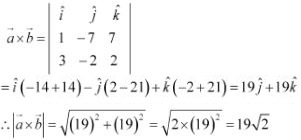2. Find a unit vector perpendicular to each of the vector a+b and a-b, where a = 3i ^+2j ^+2k^ and b = i ^-2j ^-2k^ .

Solution

We have,
a = 3i ^+2j ^+2k^ and b = i ^-2j ^-2k^ .
∴  a+ b = 4i ^+4j ^,  a⃗ - b = 2i + 4k^ .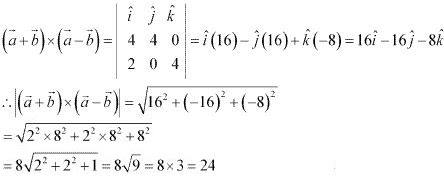Hence, the unit vector perpendicular to each of the vector a+ b and a⃗ - b, is given by the relation,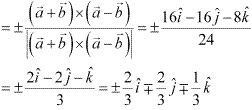3. If a unit vector a makes an angles π/3 with i ^, π/4 with j ^ and an acute angle θ with k^ , then find θ and hence, the compounds of  a⃗ .

Solution

Let unit vector  a⃗  have (a1, a2, a3) components.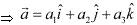Since a is a unit vector, |a| = 1.
Also, it is given that a makes angles π/3 with i ^, π/4 with j and an acute angle θ with k^ .
Then, we have :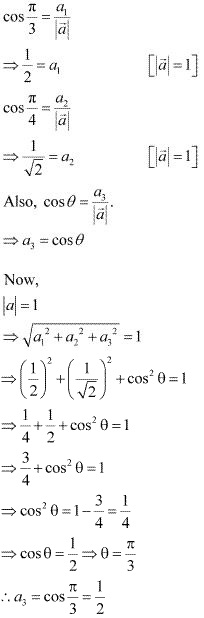Hence, θ = π/3 and the components of a are (1/2, 1/√2, 1/2).

4. Show that (a⃗ -  b) × (a+ b) = 2(a⃗ × b)

Solution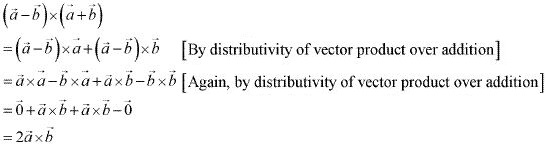5. Find λ and μ if (2i ^-6j ^-27k^) × (i ^+ λj ^- μk^) = 0⃗ .
Solution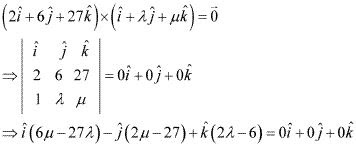On comparing the corresponding components, we have :
6μ - 27λ = 0
2μ - 27 = 0
2λ - 6 = 0
Now,
2λ - 6 = 0
⇒ λ = 3
2μ - 27 = 0
⇒ μ = 27/2
Hence, λ = 3 and μ = 27/2.

6. Given that a⃗ . b⃗ = 0 and a× b = 0. What can you conclude about the vectors a⃗ and b⃗ .
Solution
a⃗ . b⃗ = 0
Then,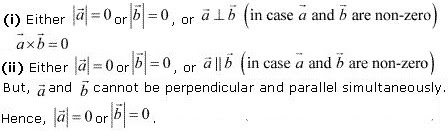7. Let the vectors a⃗ , b⃗ , c⃗ = given as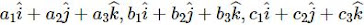. Then show that = a×(b⃗ + c) = a×b⃗ + a×c⃗ .
Solution
We have,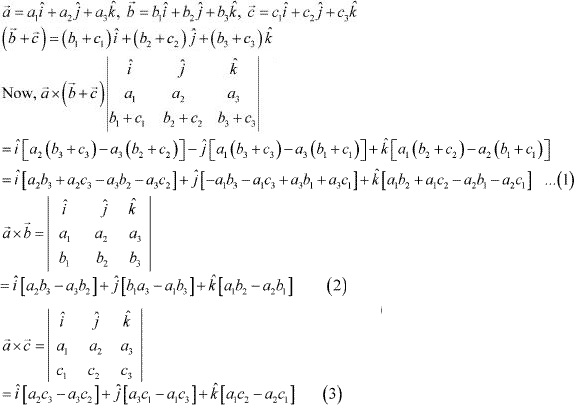On adding (2) and (3), we get :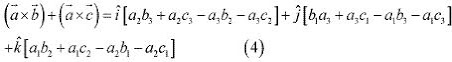Now, from (1) and (4), we have :
a×(b⃗ + c) = a⃗ × b⃗ +  a× c
Hence, the given result is proved.

8. If either a⃗ = 0⃗ or b⃗ = 0 then a⃗ × b⃗ = 0. Is the converse true? Justify your answer with an example.
Solution
Take any parallel non - zero vectors so that a⃗ × b⃗ = 0⃗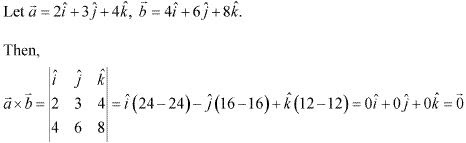It can now be observed that :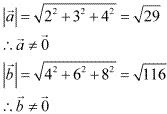Hence, the converse of the given statement need not be true.

9. Find the area of the triangle with vertices A (1, 1, 2), B (2, 3, 5) and C (1, 5, 5).
Solution
The vertices of triangle ABC are given as  A (1, 1, 2), B (2, 3, 5) and C (1, 5, 5).
The adjacent sides AB⃗ ×BC of ΔABC are given as :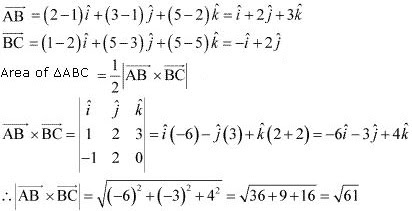Hence, the area of ΔABC is √61/2 square units.

10. Find the area of the parallelogram whose adjacent sides are determined by the vector a = i ^- j ^+3k^ and b = 2i ^-7j ^+k^ .

Solution

The area of the parallelogram whose adjacent sides are a⃗ and b⃗ is | a× b|.

Adjacent sides are given as :
a = i - j ^ +3k^  and b = 2i - 7j ^ + k^  .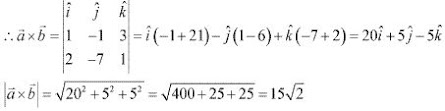Hence, the area of the given parallelogram is 15√2 square units.

11. Let the vectors a⃗ and b be such that |a| = 3 and |b| = √2/3, then a⃗ × b is a unit vector, if the angle between a⃗ and b is:
(A) π/6
(B) π/4
(C) π/3
(D) π/2

Solution

It is given that |a| = 3 and |b| = √2/3.
We know that a×b⃗ = |a||b| sinθ n ^, where n is a unit vector perpendicular to both a⃗ and b⃗ θ is the angle between a⃗ and b⃗
Now, a⃗ × b⃗ is a unit vector if |a⃗ × b| = 1.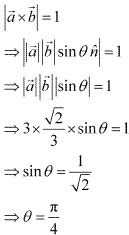Hence, a⃗ × b⃗ is a unit vector if the angle between a⃗ and b⃗ is π/4.

12. Area of a rectangle having vertices, A, B, C and D with position vectors -i ^+ (1/2)j ^+ 4k^ ,  i ^+(1/2)j ^ +4k^ , and -i ^-(1/2)j ^+4k^ and respectively is
(A) 1/2
(B) 1
(C) 2
(D) 4

Solution

The position vectors of vertices A, B, C,, and D of rectangle ABCD are given as :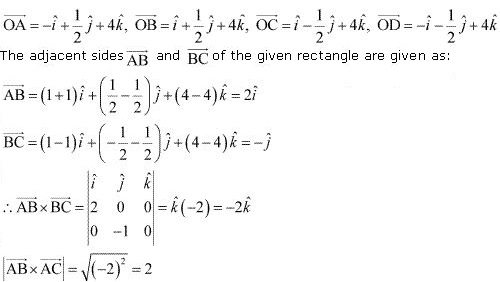Now, it is known that the area of a parallelogram whose adjacent sides are a⃗ and b⃗ is |a×b|.
Hence, the area of the given rectangle is |AB×BC| = 2 square units.